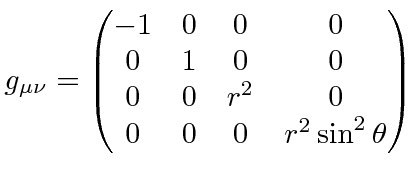## The Metric Tensor

In the Riemannian geometry of General Relativity, lengths (dot products) are computed using a metric tensor which depends on the stress-energy tensorin Einstein's equation. In flat Minkowski space:This could also be written in spherical coordinates.In General Relativity, the metric tensor can be changed significantly by large masses and also can get components off the diagonal.

The usual way to keep track of dot products etc. is to introduce upper and lower indices on vectors (and tensors). A dot product is defined to be between one vector with a lower index and another with an upper index.(Our usual vector is the one with the upper index.) An index can be lowered by using the metric tensor.This introduces the minus sign into the time component of the dot product. In general relativity, it changes how we measure 4D lengths in the region of masses.

The simplest example is the solution of the Einstein equations by Schwarzschild for problems with spherical symmetry.

Jim Branson 2012-10-21